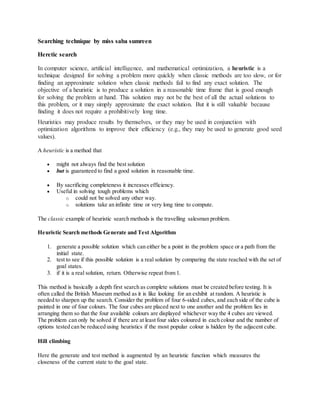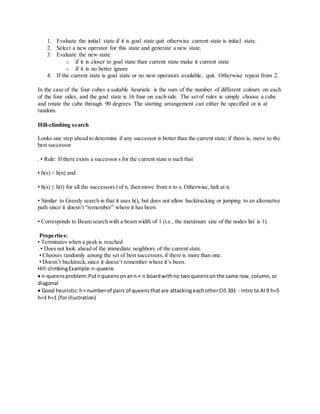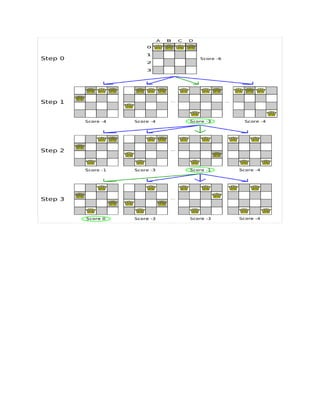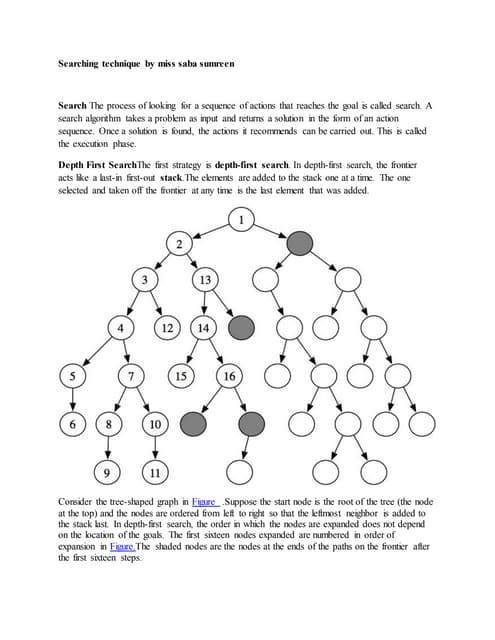AnúncioPróximos SlideSharessearching technique
Carregando em ... 3
1 de 3
Anúncio

### searching

1. Searching technique by miss saba sumreen Heretic search In computer science, artificial intelligence, and mathematical optimization, a heuristic is a technique designed for solving a problem more quickly when classic methods are too slow, or for finding an approximate solution when classic methods fail to find any exact solution. The objective of a heuristic is to produce a solution in a reasonable time frame that is good enough for solving the problem at hand. This solution may not be the best of all the actual solutions to this problem, or it may simply approximate the exact solution. But it is still valuable because finding it does not require a prohibitively long time. Heuristics may produce results by themselves, or they may be used in conjunction with optimization algorithms to improve their efficiency (e.g., they may be used to generate good seed values). A heuristic is a method that  might not always find the best solution  but is guaranteed to find a good solution in reasonable time.  By sacrificing completeness it increases efficiency.  Useful in solving tough problems which o could not be solved any other way. o solutions take an infinite time or very long time to compute. The classic example of heuristic search methods is the travelling salesman problem. Heuristic Search methods Generate and Test Algorithm 1. generate a possible solution which can either be a point in the problem space or a path from the initial state. 2. test to see if this possible solution is a real solution by comparing the state reached with the set of goal states. 3. if it is a real solution, return. Otherwise repeat from 1. This method is basically a depth first search as complete solutions must be created before testing. It is often called the British Museum method as it is like looking for an exhibit at random. A heuristic is needed to sharpen up the search. Consider the problem of four 6-sided cubes, and each side of the cube is painted in one of four colours. The four cubes are placed next to one another and the problem lies in arranging them so that the four available colours are displayed whichever way the 4 cubes are viewed. The problem can only be solved if there are at least four sides coloured in each colour and the number of options tested can be reduced using heuristics if the most popular colour is hidden by the adjacent cube. Hill climbing Here the generate and test method is augmented by an heuristic function which measures the closeness of the current state to the goal state.
2. 1. Evaluate the initial state if it is goal state quit otherwise current state is initial state. 2. Select a new operator for this state and generate a new state. 3. Evaluate the new state o if it is closer to goal state than current state make it current state o if it is no better ignore 4. If the current state is goal state or no new operators available, quit. Otherwise repeat from 2. In the case of the four cubes a suitable heuristic is the sum of the number of different colours on each of the four sides, and the goal state is 16 four on each side. The set of rules is simply choose a cube and rotate the cube through 90 degrees. The starting arrangement can either be specified or is at random. Hill-climbing search Looks one step ahead to determine if any successor is better than the current state; if there is, move to the best successor . • Rule: If there exists a successor s for the current state n such that • h(s) < h(n) and • h(s) ≤ h(t) for all the successors t of n, then move from n to s. Otherwise, halt at n. • Similar to Greedy search in that it uses h(), but does not allow backtracking or jumping to an alternative path since it doesn’t “remember” where it has been. • Corresponds to Beam search with a beam width of 1 (i.e., the maximum size of the nodes list is 1). Properties: • Terminates when a peak is reached . • Does not look ahead of the immediate neighbors of the current state. • Chooses randomly among the set of best successors,if there is more than one. • Doesn’t backtrack,since it doesn’t remember where it’s been. Hill-climbingExample:n-queens  n-queensproblem:Putnqueensonann × n boardwithno twoqueensonthe same row, column,or diagonal  Good heuristic:h= numberof pairs of queensthatare attackingeachotherCIS 391 - Intro to AI9 h=5 h=3 h=1 (forillustration)
Anúncio